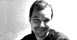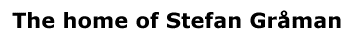Since 12 August, 2008.# Glossary

Bosons
All known particles are either bosons or fermions. Bosons have integral quantum numbers of spin: 0,1,2,etc. They obey Bose statistics in that an arbitrary number bosons can occupy the same quantum state. Examples of bosons are the photon, graviton, W and Z.
Electromagnetic force
One of the four fundamental forces it includes the familiar forces of electricity and magnetism. It is described by a U(1) gauge theory called "quantum electrodynamics" (QED). It is mediated by the photon.
Electroweak force
The electromagnetic and weak forces are known to unify into one electroweak force. It is also known as SU(2)xU(1).
Fermions
All known particles are either fermions or bosons. Fermions have half-integral quantum numbers of spin: 1/2, 3/2, etc. They obey the Pauli exclusion principle in that no two fermions can occupy the same quantum state. Examples of fermions are the electron, proton, neutron, and quark.
Gauge bosons
Particles which mediate interactions associated with gauge symmetries. They have spin 1 and are sometimes called  vector bosons. In the standard model the gauge bosons are the gluons, photons, W and Z.
Gauge theory
Most of the successful models of particle physics are based on the concept of a gauge symmetry.  Various fields in such a theory can transform into each other.  The allowed gauge transformations are dictated by a set of the rules which form the gauge group of the theory. When we can make different gauge transformations at arbitrary points in spacetime, and the theory is unchanged by these transformations, then the theory has a local gauge symmetry.
GeV
Short for "Giga electron volts" which means one billion electron volts.  An electron volt is the amount of energy required to move a single electron through a 1 volt potential.  The rest energy of an electron is about 0.0005 GeV.  The rest energy of a proton is about 0.938 GeV.  The beam energies of the world's largest particle accelerators reach about 1000 GeV.  To probe the string scale directly we would need accelerators which could read energies of 10^18 GeV.
Gravity
One of the four fundamental forces, gravity is the weakest force but it is always attractive and has an infinite range. Therefore its effects are cumulative and it is the dominant force for large scale structures in the universe such as solar systems, galaxies, and clusters. Einstein's theory of general relativity is a classical theory of gravitation. One of the major triumphs of superstring theory is that it is a consistent theory of quantum gravity (which contains general relativity in a low energy limit). Gravity is mediated by the spin 2 graviton.
SO(N)
The group of N x N orthogonal matrices with determinant equal to one.  Orthogonal means that the transpose (switching rows and columns) of the matrix equals the inverse.
Spacetime
We are used to thinking of our world as having 3 space dimensions and 1 time dimension. From the theory of special relativity we know that space and time are intimately linked. Therefore we combine them into a 4-dimensional spacetime (or 3+1 dimensional spacetime to be precise). Then the trajectory of a moving particle in this spacetime is an invariant object called a 'worldline'. How we measure the space and time dimensions amounts to a preferred set of coordinate axes which depends on our frame of reference. We generalize to higher dimensional spacetimes simply by adding more space dimensions (e.g. 10-dimensional spacetime has 9 space dimensions and 1 time dimension).
Standard Model
An ad hoc but amazingly successfull collection of theories that describes the four fundamental forces at energies currently accessible to experiments. The theories are the SU(2) x U(1) electroweak theory, the SU(3) (QCD) theory of strong interactions and the classical theory of general relativity for gravitation.  The various particles and interactions are summarized in this diagram from the Particle Data Group. Visit CPEP's The Particle Adventure for more information.
Strong force
One of the four fundamental forces, the strong force is responsible for binding quarks into nucleons like the proton and neutron. The strong force actually gets weaker at high energies. It is also known as the color force. It is described by an SU(3) gauge theory called "quantum chromodynamics" (QCD). It is mediated by gluons.
SU(N)
The group of N x N unitary matrices with determinant equal to one.  Unitary means that the adjoint (complex conjugate transpose) of the matrix equals the inverse.
Supersymmetry
A theory which unifies bosons and fermions. Every known particle would be paired with a "superpartner" of the opposite type (boson vs. fermions). The major goal of the newest high energy accelerators is to discover these superpartners and to find evidence for supersymmetry. It is an essential ingredient in realistic string theory models, hence the "super" in "superstring".
Supergravity
A special kind supersymmetric of point particle quantum field theory, in which the graviton is in a supermultiplet. The graviton is a boson with spin 2, and there is generically a fermionic superpartner with spin 3/2 called a "gravitino"
S-Duality
This refers to the situation where the strong coupling limit of one theory is described by a different theory at weak coupling. When both theories are at weak coupling they may look very different, however when one is at strong coupling and the other at weak coupling they describe exactly the same physics.
T-Duality
This refers to the situation where one string theory compactified on a circle of radius R, and another string theory compactified on circle of radius 1/R describe the same physics. Therefore when one of the theories is on a very small circle the other theory is on a very large circle.
Weak force
One of the four fundamental forces, it is responsible for weak interactions like radioactive beta decay (neutron -> proton + electron + neutrino). It is mediated by W and Z bosons and is described by a spontaneously broken SU(2) gauge theory.

| Contact and Feedback |